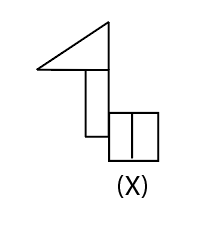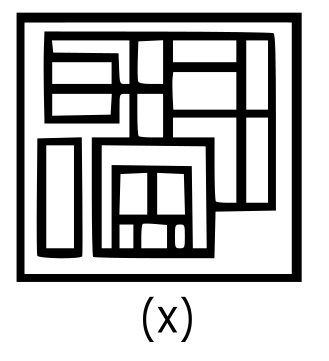# Delhi SI CPO Nov 23 Shift-2

Instructions

For the following questions answer them individually

Question 1

# If ‘A’ denotes‘ addition’, ‘B’ denotes ‘multiplication’, ‘C’ denotes ‘subtraction’, and ‘D’ denotes ‘division’, then what will be the value of the following expression?74 A(31 B 2) B 2 C (68 C 4) D (4 B 2) = ?Question 2

# Select the option in which the given figure (X) is embedded in the (rotation is NOT allowed)Question 3

# At the time of sunrise, Diksha and Arpita are sitting in a park with their backs facing each other. If the shadow of Diksha falls to the right ofArpita, then in which direction is Arpita facing?Question 4

# Select the correct combination of mathematical signs that can sequentially replace the * signs from left to right to balance the following equation.$$24 * 12 * 35 * 24 * 6 * 319$$Question 5

# Three of the following four letter-clusters are alike in a certain way and oneis different. Pick the odd one out.Question 6

# Pointing to a photograph of a girl, Dev said, “She is my wife’s sister’s mother’s only son’s daughter’. How is Dev’s wife related to that girl’s paternal grandfather?Question 7

# Select the letter-cluster that can replace the question mark (?) in the given letter-cluster seriesAGM, EKN, IOO, ? , UWQQuestion 8

# Select the option figure which is embedded in the figure (X) given below (rotation is NOT allowed).Question 9

# Eight friends A, B, C, D, E. F, G and H are sitting clockwise at equal distances from each other in the same sequence around circular table facing the centre. If D is facing southwest, then who is sitting third to the right of H?Question 10

# Which number will replace the question mark (?) in the following series?65, 85, 112, ? , 215, 305, 430OR